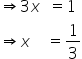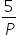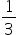Mathematics
Easy

Question

# A bag contains 5 red balls and some blue balls. If the probability of drawing a blue ball is double that of a red ball, then the number of blue balls in a bag is:

## 5101520Hint:

## The correct answer is: 10

### Let the probability of getting balls be x.Then the probability of getting blue ball = 2xAs we know the sum of probability of all the possible outcomes is 1.So, 2x + x  = 1Let total number of probability be P.The probability of getting red balls =but as derived probability of getting red ball = x =So,total number of outcome i.e. total no. balls is 15so, no. blue balls = 15 - 5 =10.#### With Turito Foundation.#### Get an Expert Advice From Turito.